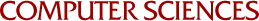Removing Randomness from Computational Number Theory

Victor Shoup
1989

In recent years, many probabilistic algorithms (i.e., algorithms that can toss coins) that run in polynomial time have been discovered for problems with no known deterministic polynomial time algorithms. Perhaps the most famous example is the problem of testing large (say, 100 digit) numbers for primality. Even for problems which are known to have deterministic polynomial time algorithms, these algorithms are often not as fast as some probabilistic algorithms for the same problem. Even though probabilistic algorithms are useful in practice, we would like to know, for both theoretical and practical reasons, if randomization is really necessary to obtain the most efficient algorithms for certain problems. That is, we would like to know for which problems there is an inherent gap between the deterministic and probabilistic complexities of these problems. In this research, we consider two problems of a number theoretic nature: factoring polynomials over finite fields and constructing irreducible polynomials of specified degree over finite fields. We present new results that narrow the gap between the known deterministic and probabilistic complexities of these problems. One of our results is a deterministic polynomial time reduction from the latter problem to the former, giving rise to a deterministic algorithm for constructing irreducible polynomials that runs in polynomial time for fields of small characteristic. Another of our results is a new deterministic factoring algorithm whose worst-case running time if asymptotically faster than that of previously known deterministic algorithms for this problem. We also analyze the average-case running time of our algorithm (averaging over inputs), proving that it is just about as fast as the expected running time (averaging over coin tosses) of some of the fastest probabilistic algorithms. In particular, the average-case running time of our algorithm is polynomial.